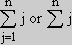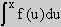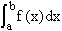18.013A | Spring 2005 | Undergraduate
Calculus with Applications

## Study Materials

### Glossary of Notations

NOTATION MEANING
i The square root of minus one
f(x) The value of the function f at argument x
sin(x) The value of the sine function at argument x
exp(x) The value of the exponential function at argument x. This is often written as ex
a^x The number a raised to the power x; for rational x is defined by inverse functions
ln x The inverse function to exp x
ax Same as a^x
logba The power you must raise b to in order to get a; $$b^{\log_{b}a} = a$$
cos x The value of the cosine function (complement of the sine) at argument x
tan x Works out to be sin x/cos x
cot x The value of the complement of the tangent function or cos x/sin x
sec x Value of the secant function, which turns out to be 1/cos x
csc x Value of the complement of the secant, called the cosecant. It is 1/sin x
asin x The value, y, of the inverse function to the sine at argument x. Means x = sin y
acos x The value, y, of the inverse function to cosine at argument x. Means x = cos y
atan x The value, y, of the inverse function to tangent at argument x. Means x = tan y
acot x The value, y, of the inverse function to cotangent at argument x. Means x = cot y
asec x The value, y, of the inverse function to secant at argument x. Means x = sec y
acsc x The value, y, of the inverse function to cosecant at argument x. Means x = csc y
θ A standard symbol for angle. Measured in radians unless stated otherwise. Used especially for atan x/y when x, y, and z are variables used to describe point in three dimensional space
i, j, k Unit vectors in the x y and z directions respectively
(a, b, c) A vector with x component a, y component b and z component c
(a, b) A vector with x component a, y component b
(a, b) The dot product of vectors a and b
a•b The dot product of vectors a and b
(a•b) The dot product of vectors a and b
|v| The magnitude of the vector v
|x| The absolute value of the number x
Σ Used to denote a summation, usually the index and often their end values are written under it with upper end value above it. For example the sum of j for j=1 to n is written as. This signifies 1 + 2 + … + n
M Used to represent a matrix or array of numbers or other entities
|v> A column vector, that is one whose components are written as a column and treated as a k by 1 matrix
<v| A vector written as a row, or 1 by k matrix
dx An “infinitesimal” or very small change in the variable x; also similarly dy, dz, dr etc…
ds A small change in distance
ρ The variable (x2 + y2 + z2)1/2 or distance to the origin in spherical coordinates
r The variable (x2 + y2)1/2 or distance to the z axis in three dimensions or in polar coordinates
|M| The determinant of a matrix M (whose magnitude is the area or volume of the parallel sided region determined by its columns or rows)
||M|| The magnitude of the determinant of the matrix M, which is a volume or area or hypervolume
det M The determinant of M
M-1 The inverse of the matrix M
v×w The vector product or cross product of two vectors, v and w
θvw The angle made by vectors v and w
A•B×C The scalar triple product, the determinant of the matrix formed by columns A, B, C
uw A unit vector in the direction of the vector w; it means the same as w/|w|
df A very small change in the function f, sufficiently small that the linear approximation to all relevant functions holds for such changes
df/dx The derivative of f with respect to x, which is the slope of the linear approximation to f
f ’ The derivative of f with respect to the relevant variable, usually x
∂f/∂x The partial derivative of f with respect to x, keeping y, and z fixed. In general a partial derivative of f with respect to a variable q is the ratio of df to dq when certain other variables are held fixed. Where there is possible misunderstanding over which variables are to be fixed that information should be made explicit
(∂f/∂x)|r,z The partial derivative of f with respect to x keeping r and z fixed
grad f The vector field whose components are the partial derivatives of the function f with respect to x, y and z: [(∂f/∂x), (∂f/∂y), (∂f/∂z)] or (∂f/∂x)i + (∂f/∂y)j + (∂f/∂z)k; called the gradient of f
The vector operator (∂/∂x)i + (∂/∂x)j + (∂/∂x)k, called “del”
∇f The gradient of f; its dot product with uw is the directional derivative of f in the direction of w
∇•w The divergence of the vector field w; it is the dot product of the vector operator ∇ with the vector w, or (∂wx /∂x) + (∂wy /∂y) + (∂wz /∂z)
curl w The cross product of the vector operator ∇ with the vector w
∇×w The curl of w, with components [(∂fz /∂y) - (∂fy /∂z), (∂fx /∂z) - (∂fz /∂x), (∂fy /∂x) - (∂fx /∂y)]
∇•∇ The Laplacian, the differential operator: (∂2/∂x2) + (∂/∂y2) + (∂/∂z2)
f “(x) The second derivative of f with respect to x; the derivative of f ‘(x)
d2f/dx2 The second derivative of f with respect to x
f(2)(x) Still another form for the second derivative of f with respect to x
f(k)(x) The k-th derivative of f with respect to x; the derivative of f(k-1) (x)
T Unit tangent vector along a curve; if curve is described by r(t), T = (dr/dt)/|dr/dt|
ds A differential of distance along a curve
κ The curvature of a curve; the magnitude of the derivative of its unit tangent vector with respect to distance on the curve: |dT/ds|
N A unit vector in the direction of the projection of dT/ds normal to T
B A unit vector normal to the plane of T and N, which is the plane of curvature
τ The torsion of a curve; |dB/ds|
g The gravitational constant
F The standard symbol for force in mechanics
k The spring constant of a spring
pi The momentum of the i-th particle
H The Hamiltonian of a physical system, which is its energy expressed in terms of {ri} and {pi}, position and momentum
{Q, H} The Poisson bracket of Q and HAn antiderivative of f(x) expressed as a function of xThe definite integral of f from a to b. When f is positive and a < b holds, then this is the area between the x axis the lines y = a, y = b and the curve that represents the function f between these lines
L(d) A Riemann sum with uniform interval size d and f evaluated at the left end of each subinterval
R(d) A Riemann sum with uniform interval size d and f evaluated at the right end of each subinterval
M(d) A Riemann sum with uniform interval size d and f evaluated at the maximum point of f in each subinterval
m(d) A Riemann sum with uniform interval size d and f evaluated at the minimum point of f in each subinterval